Select Page

# Maths Vector Algebra CBSE 12 Science MCQ in English Solutions

Maths Vector Algebra CBSE 12 Science MCQ in English Solutions to enable students to get Solutions in a narrative video format for the specific question.

Expert Teacher provides Maths Vector Algebra CBSE 12 Science MCQ English Solutions. This video solution will be useful for students to understand how to write an answer in exam in order to score more marks. This teacher uses a narrative style for a question from Vector Algebra not only to explain the proper method of answering question, but deriving right answer too.

Please find the question below and view the Solution in a narrative video format.

Question:

Solution Video in English:

You can select video Solutions from other languages also. Please check Solutions in ( Hindi )

## Similar Questions from CBSE, 12th Science, Maths, Vector Algebra

Question 1 :  Find the sum of the vectors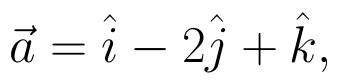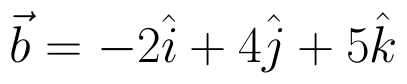and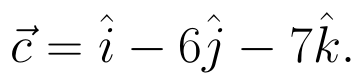(View Answer Video)

Question 2 : Find the area of the triangle with vertices A(1, 1, 2), B(2, 3, 5) and C(1, 5, 5). (View Answer Video)

Question 3 : Find the projection of the vector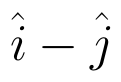on the vector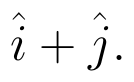(View Answer Video)

Question 4 : Find the vector quantities from the following:
(i) Time period          (ii) Distance          (iii) Force
(iv) Velocity               (v) Work done

Question 5 : Find the magnitude of two vectors a and b having the same magnitude and such that the angle between them is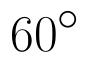and their scalar product is 1/2. (View Answer Video)

### Three Dimensional Geometry

Question 1 : If a line has the direction ratios -18, 12, -4, then what are its direction cosines? (View Answer Video)

Question 2 : Find the angle between the planes 7x + 2y + 6z = 15 and 3x - y + 10z = 17. (View Answer Video)

Question 3 : Write the vector equation of a line passing through the point (1, -1, 2) and parallel to the line whose equations are,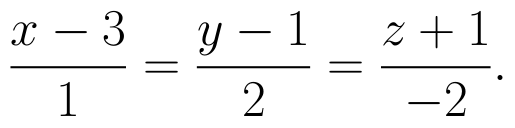(View Answer Video)

Question 4 : Find the equation of the plane passing through the line of intersection of the planes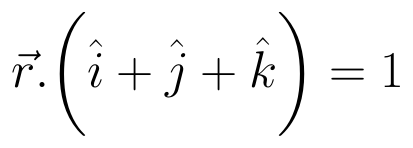and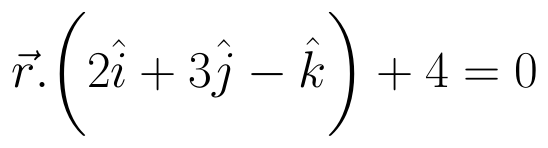which is perpendicular to the plane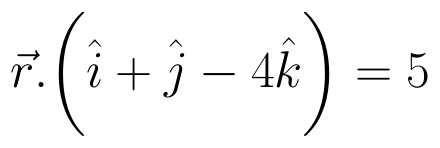. (View Answer Video)

Question 5 : Find the vector equation of the plane which contains the line of intersection of the planes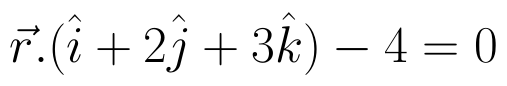and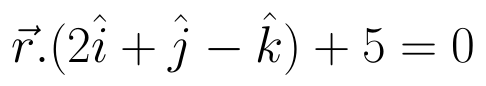and which is perpendicular to the plane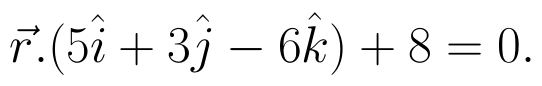(View Answer Video)

### Inverse Trigonometric Functions

Question 1 : Write the principal value of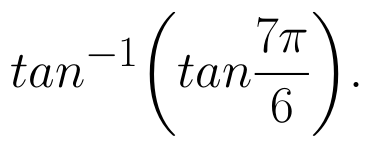(View Answer Video)

Question 2 :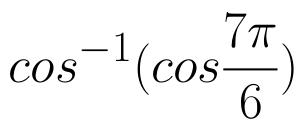is equal to : (View Answer Video)

Question 3 : Solve for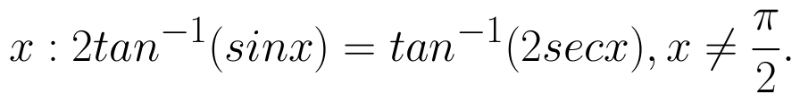(View Answer Video)

Question 4 :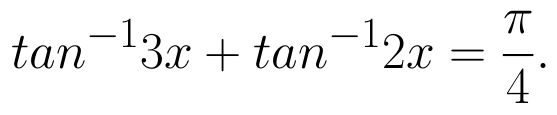What value do you observe in real life scenario ? (View Answer Video)

Question 5 : Write the principal value of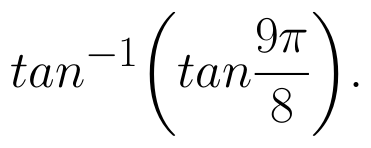(View Answer Video)

### Continuity and Differentiability

Question 1 : If x and y are connected parametrically by the equation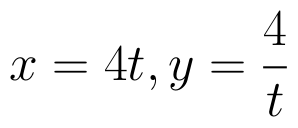, without eliminating the parameter, find. (View Answer Video)

Question 2 : Differentiate w.r.t.x the function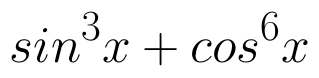. (View Answer Video)

Question 3 :  Find the second order derivative of the function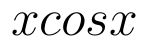. (View Answer Video)

Question 4 : Findfor the function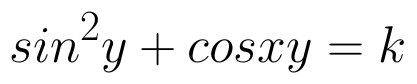. (View Answer Video)

Question 5 : Differentiate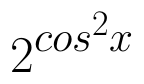w.r.t.x. (View Answer Video)